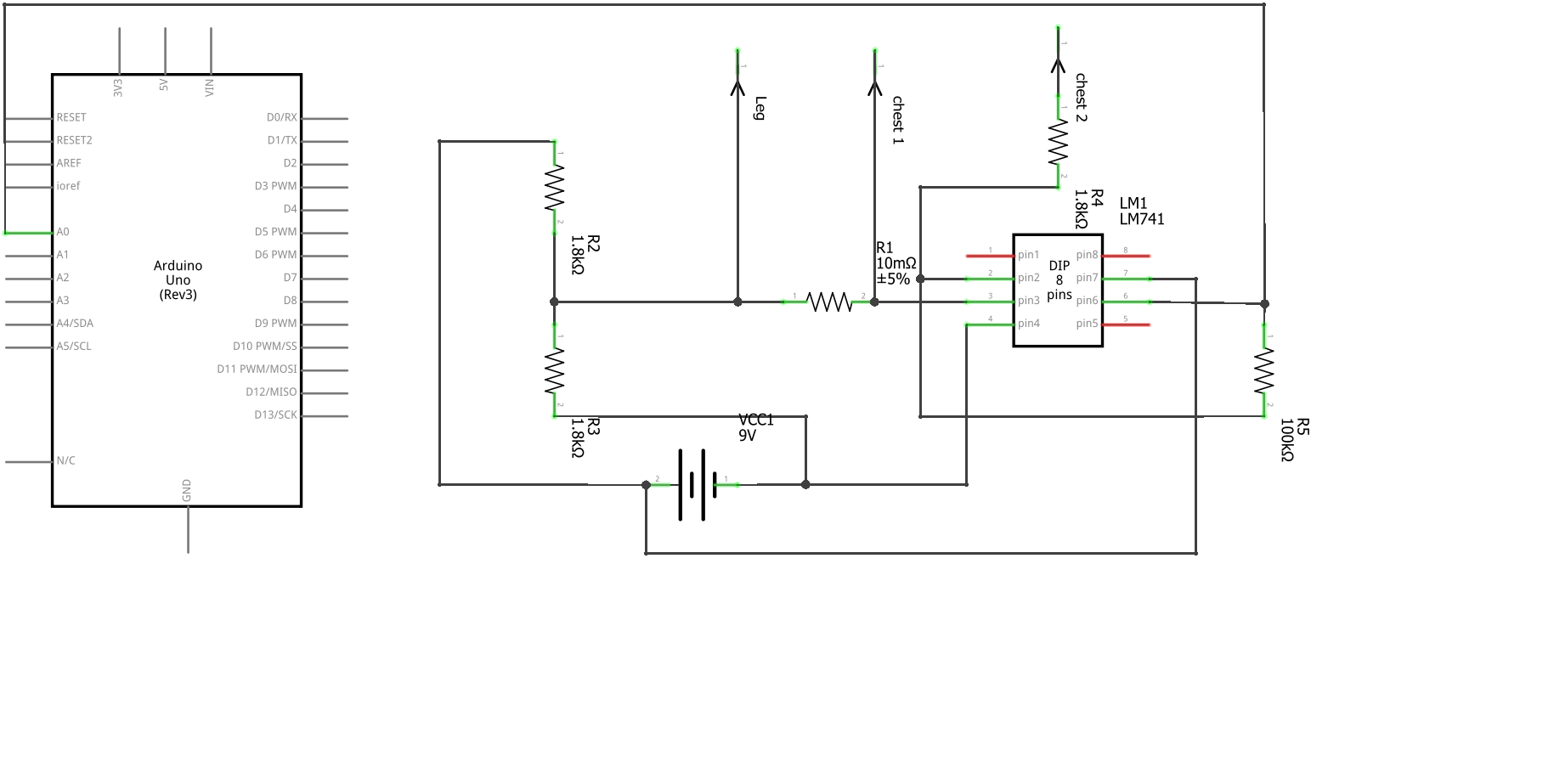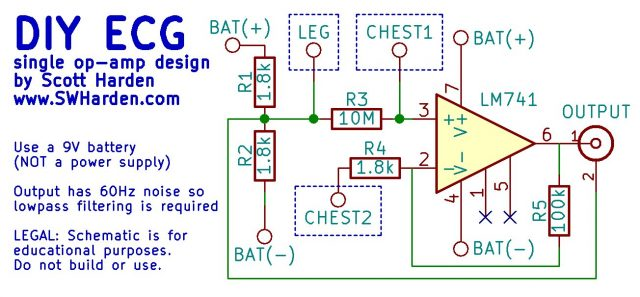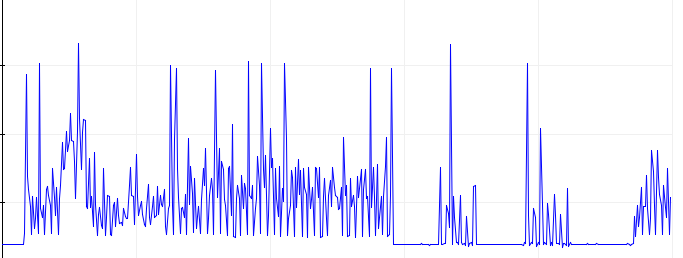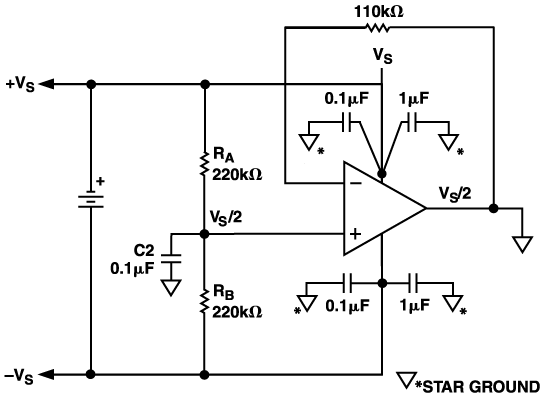# Another ECG Question thread (Using FFT, low pass filter)

I'll try to avoid the pitfalls and failings of other people posting questions about ECG/EKG projects! Thus, this amount of data may be overkill.

This is based on this project: DIY ECG with 1 op-amp

I'll go in to more detail below, but, what I'm trying to figure out is how to:

1. Get a clean plot of the heartbeat in at least the serial plotter as demonstrated in the code running in python on my PC
2. Detect the highest point of the heartbeat in order to count beats per minute (Reach goal!)

First, the circuit:As you can see, it's very similar to the one used in the SWHarden example:But instead of a headphone jack, I'm plugging it into A0 on an Arduino Uno, and connecting ground to.... well, ground!

It's still powered off of a 9 volt because I'd rather not be electrocuted. (please excuse the messy Fritzing, I had to download it and mess with it for the first time, for this question)

"How do you know the circuit you're using is working?"

Because when I run SWHarden's code on my computer, it generates this:

(P.S. Notice the 49hz low pass filter there)

My first attempt to just take a look at what was coming out of the circuit on my arduino was this code:

``````#include <FilterOnePole.h>

float filterFrequency =49.0  ;

// create a one pole (RC) lowpass filter
FilterOnePole lowpassFilter( LOWPASS, filterFrequency );
void setup() {
Serial.begin(115200);

}

// the loop routine runs over and over again forever:

void loop() {
// read the input on analog pin 0:

int sensorValue = lowpassFilter.input(  analogRead(A0) );
// print out the value you read:

// Serial.println(sensorValue);
//delayMicroseconds(3900);
//delayMicroseconds(125);
Serial.println(sensorValue);

}
``````

That low pass filter is me trying to deal with house current interference.

On the serial plotter:

notice that dipped bit there? It was consistent with my heartbeat, so something is getting through.

Now, bear with me here. This is the important part of SWHarden's Python code, I think:

``````def FFT(data,rate):
"""given some data points and a rate, return [freq,power]"""
data=data*np.hamming(len(data))
fft=np.fft.fft(data)
fft=10*np.log10(np.abs(fft))
freq=np.fft.fftfreq(len(fft),1/rate)
return freq[:int(len(freq)/2)],fft[:int(len(fft)/2)]
``````
``````def softEdges(self,data,fracEdge=.05):
"""multiple edges by a ramp of a certain percentage."""
rampSize=int(len(data)*fracEdge)
mult = np.ones(len(data))
window=np.hanning(rampSize*2)
mult[:rampSize]=window[:rampSize]
mult[-rampSize:]=window[-rampSize:]
return data*mult

def getFiltered(self,freqHighCutoff=50):
if freqHighCutoff<=0:
return self.data
fft=np.fft.fft(self.softEdges(self.data)) #todo: filter needed?
trim=len(fft)/self.rate*freqHighCutoff
fft[int(trim):-int(trim)]=0
return np.real(np.fft.ifft(fft))
``````

It appears they're defining a function to use FFT to find the dominant signal? Given a sampling rate?
And then they're.... softening the edges? That's starting to get well outside my knowledge.

I tried to get something rolling with example FFT Code for arduino but it's more or less a disaster.

``````#include "arduinoFFT.h"

#define SAMPLES 128             //Must be a power of 2
#define SAMPLING_FREQUENCY 44100 //Hz, must be less than 10000 due to ADC
//#define SAMPLING_FREQUENCY 256 //Hz, must be less than 10000 due to ADC
#include <FilterOnePole.h>
arduinoFFT FFT = arduinoFFT();
float filterFrequency = 49.0  ;
// create a one pole (RC) lowpass filter
FilterOnePole lowpassFilter( LOWPASS, filterFrequency );
unsigned int sampling_period_us;
unsigned long microseconds;

double vReal[SAMPLES];
double vImag[SAMPLES];

void setup() {
Serial.begin(115200);
pinMode(LED_BUILTIN, OUTPUT);
sampling_period_us = round(1000000 * (1.0 / SAMPLING_FREQUENCY));
digitalWrite(LED_BUILTIN, LOW);
}

void loop() {

/*SAMPLING*/
for (int i = 0; i < SAMPLES; i++)
{
microseconds = micros();    //Overflows after around 70 minutes!

vImag[i] = 0;

while (micros() < (microseconds + sampling_period_us)) {
}
}

/*FFT*/
FFT.Windowing(vReal, SAMPLES, FFT_WIN_TYP_HAMMING, FFT_FORWARD);
FFT.Compute(vReal, vImag, SAMPLES, FFT_FORWARD);
FFT.ComplexToMagnitude(vReal, vImag, SAMPLES);
double peak = FFT.MajorPeak(vReal, SAMPLES, SAMPLING_FREQUENCY);

/*PRINT RESULTS*/
Serial.println(peak);     //Print out what frequency is the most dominant.

for (int i = 0; i < (SAMPLES / 2); i++)
{
/*View all these three lines in serial terminal to see which frequencies has which amplitudes*/
//  Serial.println((i * 1.0 * SAMPLING_FREQUENCY) / SAMPLES, 1);
// Serial.print(" ");
//   Serial.println(vReal[i], 1);    //View only this line in serial plotter to visualize the bins
}

delayMicroseconds(125);

}
``````

That code in the plotter does this:Those totally empty spots are where I unplugged stuff to make sure it was "sensing" something at all.

Okay, with all of that madness behind us, what I'm trying to figure out the meaning of the FFT code SWharden has there.

Am I sampling at the wrong rate? Do I need to set my arduino's processor to a particular speed so I can sample appropriately? I have a feeling this is some kind of mismatch between frequency and sampling but I could be totally wrong. I suppose I'm looking for a direction to go with my research. Why do I need FFT in order to get that clean EKG signal?

Any help is appreciated, thanks! I'll do my best to do my own research but I've read through a lot of failed threads, and a lot of other code (mostly based on the LED based heartbeat modules) and none of it seemed to lead me in the correct direction.

Thanks for sticking with me through all of that, and again, thanks for any help!

Adding a more accurate schematic reflecting the ground connection, since moving that to various places on the op-amp circuit seems to very much affect the signal. (been moving it between the ground from the battery, and the "ground" that's going to the output

The op amp circuit lacks the essential decoupling capacitors (minimum, 0.1 uF directly across the op amp power pins and 0.1 uF from the center tap of the two 1.8K resistors to the negative battery terminal), similar to the image below.The circuit should be contained in a metal box, with the box grounded to the Arduino ground, to reduce AC hum pickup.

Finally, you can make the op amp act as a first order low pass filter by adding a capacitor across the feedback resistor R5. 1 uF/100K would lead to a 0.1 s time constant, substantially reducing AC hum.

jremington:
The op amp circuit lacks the essential decoupling capacitors (minimum, 0.1 uF directly across the op amp power pins and 0.1 uF from the center tap of the two 1.8K resistors to the negative battery terminal), similar to the image below.The circuit should be contained in a metal box, with the box grounded to the Arduino ground, to reduce AC hum pickup.

Finally, you can make the op amp act as a first order low pass filter by adding a capacitor across the feedback resistor R5. 1 uF/100K would lead to a 0.1 s time constant, substantially reducing AC hum.

Awesome! Thank you. I'll give it a shot!

Is the python code compensating for this lack of decoupling caps in the code? Is that why it appears to be working?

I'll still give this capacitor thing a shot and report back. Thanks again!

Without the decoupling capacitors, the op amp could be simply oscillating.

I'm not convinced that anything is "working", and your code will never, ever work if you ignore comments as cavalierly as you do in the following:

``````#define SAMPLING_FREQUENCY 44100 //Hz, must be less than 10000 due to ADC
``````

If you want FFT code that runs at a reasonable sampling rate, use the Open Music Labs code. But you don't need high sampling frequencies for a heart rate monitor. 200 Hz should be plenty fast.

jremington:
The op amp circuit lacks the essential decoupling capacitors (minimum, 0.1 uF directly across the op amp power pins and 0.1 uF from the center tap of the two 1.8K resistors to the negative battery terminal), similar to the image below.The circuit should be contained in a metal box, with the box grounded to the Arduino ground, to reduce AC hum pickup.

Finally, you can make the op amp act as a first order low pass filter by adding a capacitor across the feedback resistor R5. 1 uF/100K would lead to a 0.1 s time constant, substantially reducing AC hum.

I tried the caps, though they were a bit bigger than .1 uF... they were 4.7uF as that's all I had at the bench. I can get the smaller caps in the morning but I wanted to give this a shot. If the caps are too big, and that's what is causing this latest issue, whoops!

Anyhow, here's the graph of the output from the circuit with caps. Notice that it's no longer the nice clean EKG I was getting before:(for reference, this is what that graph typically looks like on the PC)

(the graph on the PC seems to work, as it matches my heartbeat, and has that built in low pass filter)

jremington:
Without the decoupling capacitors, the op amp could be simply oscillating.

I'm not convinced that anything is "working", and your code will never, ever work if you ignore comments as cavalierly as you do in the following:

``````#define SAMPLING_FREQUENCY 44100 //Hz, must be less than 10000 due to ADC
``````

If you want FFT code that runs at a reasonable sampling rate, use the Open Music Labs code. But you don't need high sampling frequencies for a heart rate monitor. 200 Hz should be plenty fast.

Oh, you're absolutely right. That bit of code modification on my part is trash. I was trying to use example FFT code in an attempt to figure out what those functions were doing. It's based on example code that's supposed to be attached to a tone generator.

I should probably just delete that bit of code because it's so bad. I was just trying to get as much information into the first post, since these EKG threads seem plagued by original poster not giving enough context.

The code that is working was written in python by SWharden. I think this comes down to, I'll need to port the code using the Arduino equivalents to the python functions. I just want to learn more about this, instead of converting one black box to another black box...if that makes any sense at all